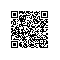# 2013-7-15学习一些修饰词的比较

//蛇形函数问题

#include<stdio.h>

#include<string.h>

void main()

{

int i = 0,n,k = 1;

printf("请输入N:");

scanf("%d",&n);  //

int j = n-1,s;

memset(s,0,sizeof(s));  //内存初始化

while(k<=n*n)

{

while(i<=n-1&&s[i][j]==0&&k<=n*n) {s[i++][j] = k++;}

i--;//不然的话上面一行i++之后越过边界了

j--;//不然会覆盖边角的那个数

while(j>=0&&s[i][j]==0&&k<=n*n)  {s[i][j--] = k++;}

j++;

i--;

while(i>=0&&s[i][j]==0&&k<=n*n)  {s[i--][j] = k++;}

i++;

j++;

while(j<=n-1&&s[i][j]==0&&k<=n*n)  {s[i][j++] = k++;}

i++;

j--;

}

//打印输出

for(int x = 0;x<n;x++)

{

for(int y = 0;y<n;y++)

{

if(s[x][y]<10)

printf("%d  ",s[x][y]);

else

printf("%d ",s[x][y]);

}

printf("\n");

}

}const和define的区别：
define不需要指名类型，const只读不能修改。const 要做类型判断

const char *p;

char const *p;

p指向可以改，*p不可改

char * const p="abc"；   *p可以改，但这儿不可改，是在静态区，初始化必须指向，不然会报错

char const * const p="abc"  ; p不可改，*p也不可改

const数据开辟在静态区，在编译的时候就开辟空间了

1.从内存上来讲  系统为指针分寸内存空间，而引用与绑定的对象共享内存空间，系统不为引用变量分配内容空间。

2指针初始化以后可以改变指向的对象，而引用定义的时候必须要初始化，且初始化以后不允许再重新绑定对象。

3.所以引用访问对象是直接访问。指针访问对象是间接访问。

4。如果pa是指针，那么*pa就是引用了。

#include<stdio.h>

void pt(int * pta,int * ptb)

{

int *ptc;

ptc=pta;pta=ptb;ptb=ptc;

}

void ref(int &ra,int &rb)

{

int rc;

rc=ra;ra=rb;rb=rc;

}

void main()

{

int a=3;int b=4;

int *pa=&a;int *pb=&b;

pt(pa,pb);

printf("zhizhen: a=%d,b=%d\n",a,b);

ref(a,b);

printf("yinyong: a=%d,b=%d\n",a,b);

}

const和static区别

const int i=10; //i不可以更改

static int a=10;//可以更改 只初始化一次

static的第二个含义是用于一个函数前，说明该函数只能在同一个文件中调用。使用钉钉扫一扫加入圈子
+ 订阅